## What Is a Confidence Interval?

A confidence interval is a type of interval estimate of a population parameter and is used to indicate the reliability of an estimate.

### Learning Objectives

Explain the principle behind confidence intervals in statistical inference

### Key Takeaways

#### Key Points

• In inferential statistics, we use sample data to make generalizations about an unknown population.
• A confidence interval is a type of estimate, like a sample average or sample standard deviation, but instead of being just one number it is an interval of numbers.
• The interval of numbers is an estimated range of values calculated from a given set of sample data.
• The principle behind confidence intervals was formulated to provide an answer to the question raised in statistical inference: how do we resolve the uncertainty inherent in results derived from data that are themselves only a randomly selected subset of a population?
• Note that the confidence interval is likely to include an unknown population parameter.

#### Key Terms

• confidence interval: A type of interval estimate of a population parameter used to indicate the reliability of an estimate.
• population: a group of units (persons, objects, or other items) enumerated in a census or from which a sample is drawn
• sample: a subset of a population selected for measurement, observation, or questioning to provide statistical information about the population

Suppose you are trying to determine the average rent of a two-bedroom apartment in your town. You might look in the classified section of the newpaper, write down several rents listed, and then average them together—from this you would obtain a point estimate of the true mean. If you are trying to determine the percent of times you make a basket when shooting a basketball, you might count the number of shots you make, and divide that by the number of shots you attempted. In this case, you would obtain a point estimate for the true proportion.

In inferential statistics, we use sample data to make generalizations about an unknown population. The sample data help help us to make an estimate of a population parameter. We realize that the point estimate is most likely not the exact value of the population parameter, but close to it. After calculating point estimates, we construct confidence intervals in which we believe the parameter lies.

A confidence interval is a type of estimate (like a sample average or sample standard deviation), in the form of an interval of numbers, rather than only one number. It is an observed interval (i.e., it is calculated from the observations), used to indicate the reliability of an estimate. The interval of numbers is an estimated range of values calculated from a given set of sample data. How frequently the observed interval contains the parameter is determined by the confidence level or confidence coefficient. Note that the confidence interval is likely to include an unknown population parameter.

### Philosophical Issues

The principle behind confidence intervals provides an answer to the question raised in statistical inference: how do we resolve the uncertainty inherent in results derived from data that (in and of itself) is only a randomly selected subset of a population? Bayesian inference provides further answers in the form of credible intervals.

Confidence intervals correspond to a chosen rule for determining the confidence bounds; this rule is essentially determined before any data are obtained or before an experiment is done. The rule is defined such that over all possible datasets that might be obtained, there is a high probability (“high” is specifically quantified) that the interval determined by the rule will include the true value of the quantity under consideration—a fairly straightforward and reasonable way of specifying a rule for determining uncertainty intervals.

Ostensibly, the Bayesian approach offers intervals that (subject to acceptance of an interpretation of “probability” as Bayesian probability) offer the interpretation that the specific interval calculated from a given dataset has a certain probability of including the true value (conditional on the data and other information available). The confidence interval approach does not allow this, as in this formulation (and at this same stage) both the bounds of the interval and the true values are fixed values; no randomness is involved.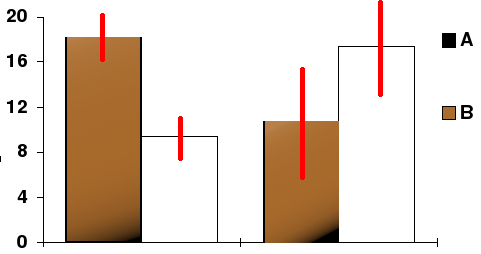Confidence Interval: In this bar chart, the top ends of the bars indicate observation means and the red line segments represent the confidence intervals surrounding them. Although the bars are shown as symmetric in this chart, they do not have to be symmetric.

## Interpreting a Confidence Interval

For users of frequentist methods, various interpretations of a confidence interval can be given.

### Learning Objectives

Construct a confidence intervals based on the point estimate of the quantity being considered

### Key Takeaways

#### Key Points

• Methods for deriving confidence intervals include descriptive statistics, likelihood theory, estimating equations, significance testing, and bootstrapping.
• The confidence interval can be expressed in terms of samples: “Were this procedure to be repeated on multiple samples, the calculated confidence interval would encompass the true population parameter 90% of the time”.
• The explanation of a confidence interval can amount to something like: “The confidence interval represents values for the population parameter, for which the difference between the parameter and the observed estimate is not statistically significant at the 10% level “.
• The probability associated with a confidence interval may also be considered from a pre- experiment point of view, in the same context in which arguments for the random allocation of treatments to study items are made.

#### Key Terms

• frequentist: An advocate of frequency probability.
• confidence interval: A type of interval estimate of a population parameter used to indicate the reliability of an estimate.

### Deriving a Confidence Interval

For non-standard applications, there are several routes that might be taken to derive a rule for the construction of confidence intervals. Established rules for standard procedures might be justified or explained via several of these routes. Typically a rule for constructing confidence intervals is closely tied to a particular way of finding a point estimate of the quantity being considered.

• Descriptive statistics – This is closely related to the method of moments for estimation. A simple example arises where the quantity to be estimated is the mean, in which case a natural estimate is the sample mean. The usual arguments indicate that the sample variance can be used to estimate the variance of the sample mean. A naive confidence interval for the true mean can be constructed centered on the sample mean with a width which is a multiple of the square root of the sample variance.
• Likelihood theory – The theory here is for estimates constructed using the maximum likelihood principle. It provides for two ways of constructing confidence intervals (or confidence regions) for the estimates.
• Estimating equations – The estimation approach here can be considered as both a generalization of the method of moments and a generalization of the maximum likelihood approach. There are corresponding generalizations of the results of maximum likelihood theory that allow confidence intervals to be constructed based on estimates derived from estimating equations.
• Significance testing – If significance tests are available for general values of a parameter, then confidence intervals/regions can be constructed by including in the $100\text{p}\%$ confidence region all those points for which the significance test of the null hypothesis that the true value is the given value is not rejected at a significance level of $1-\text{p}$.
• Bootstrapping – In situations where the distributional assumptions for the above methods are uncertain or violated, resampling methods allow construction of confidence intervals or prediction intervals. The observed data distribution and the internal correlations are used as the surrogate for the correlations in the wider population.

### Meaning and Interpretation

For users of frequentist methods, various interpretations of a confidence interval can be given:

• The confidence interval can be expressed in terms of samples (or repeated samples): “Were this procedure to be repeated on multiple samples, the calculated confidence interval (which would differ for each sample) would encompass the true population parameter 90% of the time. ” Note that this does not refer to repeated measurement of the same sample, but repeated sampling.
• The explanation of a confidence interval can amount to something like: “The confidence interval represents values for the population parameter, for which the difference between the parameter and the observed estimate is not statistically significant at the 10% level. ” In fact, this relates to one particular way in which a confidence interval may be constructed.
• The probability associated with a confidence interval may also be considered from a pre-experiment point of view, in the same context in which arguments for the random allocation of treatments to study items are made. Here, the experimenter sets out the way in which they intend to calculate a confidence interval. Before performing the actual experiment, they know that the end calculation of that interval will have a certain chance of covering the true but unknown value. This is very similar to the “repeated sample” interpretation above, except that it avoids relying on considering hypothetical repeats of a sampling procedure that may not be repeatable in any meaningful sense.

In each of the above, the following applies: If the true value of the parameter lies outside the 90% confidence interval once it has been calculated, then an event has occurred which had a probability of 10% (or less) of happening by chance.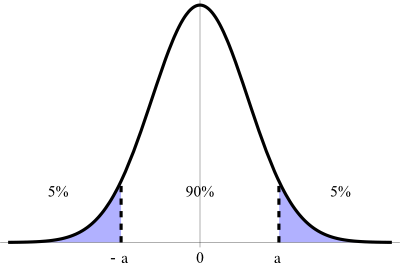Confidence Interval: This figure illustrates a 90% confidence interval on a standard normal curve.

## Caveat Emptor and the Gallup Poll

Readers of polls, such as the Gallup Poll, should exercise Caveat Emptor by taking into account the poll’s margin of error.

### Learning Objectives

Explain how margin of error plays a significant role in making purchasing decisions

### Key Takeaways

#### Key Points

• Historically, the Gallup Poll has measured and tracked the public’s attitudes concerning virtually every political, social, and economic issue of the day, including highly sensitive or controversial subjects.
• Caveat emptor is Latin for “let the buyer beware”—the property law principle that controls the sale of real property after the date of closing, but may also apply to sales of other goods.
• The margin of error is usually defined as the “radius” (or half the width) of a confidence interval for a particular statistic from a survey.
• The larger the margin of error, the less confidence one should have that the poll’s reported results are close to the “true” figures — that is, the figures for the whole population.
• Like confidence intervals, the margin of error can be defined for any desired confidence level, but usually a level of 90%, 95% or 99% is chosen (typically 95%).

#### Key Terms

• caveat emptor: Latin for “let the buyer beware”—the property law principle that controls the sale of real property after the date of closing, but may also apply to sales of other goods.
• margin of error: An expression of the lack of precision in the results obtained from a sample.

### Gallup Poll

The Gallup Poll is the division of the Gallup Company that regularly conducts public opinion polls in more than 140 countries around the world. Gallup Polls are often referenced in the mass media as a reliable and objective measurement of public opinion. Gallup Poll results, analyses, and videos are published daily on Gallup.com in the form of data-driven news.

Since inception, Gallup Polls have been used to measure and track public attitudes concerning a wide range of political, social, and economic issues (including highly sensitive or controversial subjects). General and regional-specific questions, developed in collaboration with the world’s leading behavioral economists, are organized into powerful indexes and topic areas that correlate with real-world outcomes.

### Caveat Emptor

Caveat emptor is Latin for “let the buyer beware.” Generally, caveat emptor is the property law principle that controls the sale of real property after the date of closing, but may also apply to sales of other goods. Under its principle, a buyer cannot recover damages from a seller for defects on the property that render the property unfit for ordinary purposes. The only exception is if the seller actively conceals latent defects, or otherwise states material misrepresentations amounting to fraud.

This principle can also be applied to the reading of polling information. The reader should “beware” of possible errors and biases present that might skew the information being represented. Readers should pay close attention to a poll’s margin of error.

### Margin of Error

The margin of error statistic expresses the amount of random sampling error in a survey’s results. The larger the margin of error, the less confidence one should have that the poll’s reported results represent “true” figures (i.e., figures for the whole population). Margin of error occurs whenever a population is incompletely sampled.

The margin of error is usually defined as the “radius” (half the width) of a confidence interval for a particular statistic from a survey. When a single, global margin of error is reported, it refers to the maximum margin of error for all reported percentages using the full sample from the survey. If the statistic is a percentage, this maximum margin of error is calculated as the radius of the confidence interval for a reported percentage of 50%.

For example, if the true value is 50 percentage points, and the statistic has a confidence interval radius of 5 percentage points, then we say the margin of error is 5 percentage points. As another example, if the true value is 50 people, and the statistic has a confidence interval radius of 5 people, then we might say the margin of error is 5 people.

In some cases, the margin of error is not expressed as an “absolute” quantity; rather, it is expressed as a “relative” quantity. For example, suppose the true value is 50 people, and the statistic has a confidence interval radius of 5 people. If we use the “absolute” definition, the margin of error would be 5 people. If we use the “relative” definition, then we express this absolute margin of error as a percent of the true value. So in this case, the absolute margin of error is 5 people, but the “percent relative” margin of error is 10% (10% of 50 people is 5 people).

Like confidence intervals, the margin of error can be defined for any desired confidence level, but usually a level of 90%, 95% or 99% is chosen (typically 95%). This level is the probability that a margin of error around the reported percentage would include the “true” percentage. Along with the confidence level, the sample design for a survey (in particular its sample size) determines the magnitude of the margin of error. A larger sample size produces a smaller margin of error, all else remaining equal.

If the exact confidence intervals are used, then the margin of error takes into account both sampling error and non-sampling error. If an approximate confidence interval is used (for example, by assuming the distribution is normal and then modeling the confidence interval accordingly), then the margin of error may only take random sampling error into account. It does not represent other potential sources of error or bias, such as a non-representative sample-design, poorly phrased questions, people lying or refusing to respond, the exclusion of people who could not be contacted, or miscounts and miscalculations.

### Different Confidence Levels

For a simple random sample from a large population, the maximum margin of error is a simple re-expression of the sample size $\text{n}$. The numerators of these equations are rounded to two decimal places.

• Margin of error at 99% confidence $\displaystyle \approx \frac { 1.29 }{ \sqrt { \text{n} } }$
• Margin of error at 95% confidence $\displaystyle \approx \frac { 0.98 }{ \sqrt { \text{n} } }$
• Margin of error at 90% confidence $\displaystyle \approx \frac { 0.82 }{ \sqrt { \text{n} } }$

If an article about a poll does not report the margin of error, but does state that a simple random sample of a certain size was used, the margin of error can be calculated for a desired degree of confidence using one of the above formulae. Also, if the 95% margin of error is given, one can find the 99% margin of error by increasing the reported margin of error by about 30%.

As an example of the above, a random sample of size 400 will give a margin of error, at a 95% confidence level, of $\frac{0.98}{20}$ or 0.049 (just under 5%). A random sample of size 1,600 will give a margin of error of $\frac{0.98}{40}$, or 0.0245 (just under 2.5%). A random sample of size 10,000 will give a margin of error at the 95% confidence level of $\frac{0.98}{100}$, or 0.0098 – just under 1%.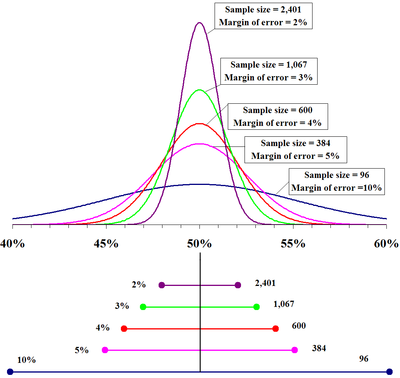Margin for Error: The top portion of this graphic depicts probability densities that show the relative likelihood that the “true” percentage is in a particular area given a reported percentage of 50%. The bottom portion shows the 95% confidence intervals (horizontal line segments), the corresponding margins of error (on the left), and sample sizes (on the right). In other words, for each sample size, one is 95% confident that the “true” percentage is in the region indicated by the corresponding segment. The larger the sample is, the smaller the margin of error is.

## Level of Confidence

The proportion of confidence intervals that contain the true value of a parameter will match the confidence level.

### Learning Objectives

Explain the use of confidence intervals in estimating population parameters

### Key Takeaways

#### Key Points

• The presence of a confidence level is guaranteed by the reasoning underlying the construction of confidence intervals.
• Confidence level is represented by a percentage.
• The desired level of confidence is set by the researcher (not determined by data ).
• In applied practice, confidence intervals are typically stated at the 95% confidence level.

#### Key Terms

• confidence level: The probability that a measured quantity will fall within a given confidence interval.

If confidence intervals are constructed across many separate data analyses of repeated (and possibly different) experiments, the proportion of such intervals that contain the true value of the parameter will match the confidence level. This is guaranteed by the reasoning underlying the construction of confidence intervals.

Confidence intervals consist of a range of values (interval) that act as good estimates of the unknown population parameter. However, in infrequent cases, none of these values may cover the value of the parameter. The level of confidence of the confidence interval would indicate the probability that the confidence range captures this true population parameter given a distribution of samples. It does not describe any single sample. This value is represented by a percentage, so when we say, “we are 99% confident that the true value of the parameter is in our confidence interval,” we express that 99% of the observed confidence intervals will hold the true value of the parameter.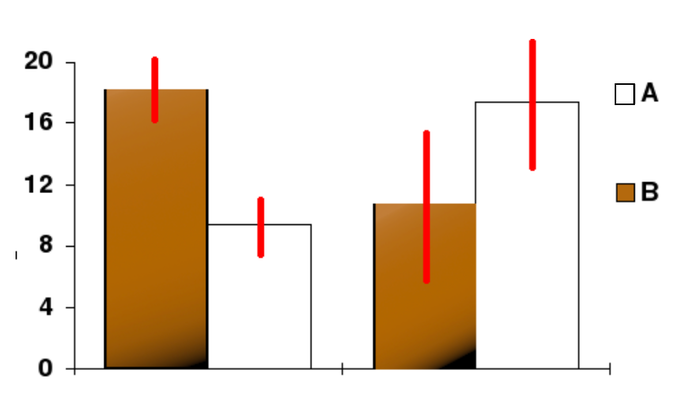Confidence Level: In this bar chart, the top ends of the bars indicate observation means and the red line segments represent the confidence intervals surrounding them. Although the bars are shown as symmetric in this chart, they do not have to be symmetric.

After a sample is taken, the population parameter is either in the interval made or not — there is no chance. The desired level of confidence is set by the researcher (not determined by data). If a corresponding hypothesis test is performed, the confidence level is the complement of respective level of significance (i.e., a 95% confidence interval reflects a significance level of 0.05).

In applied practice, confidence intervals are typically stated at the 95% confidence level. However, when presented graphically, confidence intervals can be shown at several confidence levels (for example, 50%, 95% and 99%).

## Determining Sample Size

A major factor determining the length of a confidence interval is the size of the sample used in the estimation procedure.

### Learning Objectives

Assess the most appropriate way to choose a sample size in a given situation

### Key Takeaways

#### Key Points

• Sample size determination is the act of choosing the number of observations or replicates to include in a statistical sample.
• The sample size is an important feature of any empirical study in which the goal is to make inferences about a population from a sample.
• In practice, the sample size used in a study is determined based on the expense of data collection and the need to have sufficient statistical power.
• Larger sample sizes generally lead to increased precision when estimating unknown parameters.

#### Key Terms

• law of large numbers: The statistical tendency toward a fixed ratio in the results when an experiment is repeated a large number of times.
• central limit theorem: The theorem that states: If the sum of independent identically distributed random variables has a finite variance, then it will be (approximately) normally distributed.
• Stratified Sampling: A method of sampling that involves dividing members of the population into homogeneous subgroups before sampling.

Sample size, such as the number of people taking part in a survey, determines the length of the estimated confidence interval. Sample size determination is the act of choosing the number of observations or replicates to include in a statistical sample. The sample size is an important feature of any empirical study in which the goal is to make inferences about a population from a sample.

In practice, the sample size used in a study is determined based on the expense of data collection and the need to have sufficient statistical power. In complicated studies there may be several different sample sizes involved. For example, in a survey sampling involving stratified sampling there would be different sample sizes for each population. In a census, data are collected on the entire population, hence the sample size is equal to the population size. In experimental design, where a study may be divided into different treatment groups, there may be different sample sizes for each group.

Sample sizes may be chosen in several different ways:

• expedience, including those items readily available or convenient to collect (choice of small sample sizes, though sometimes necessary, can result in wide confidence intervals or risks of errors in statistical hypothesis testing)
• using a target variance for an estimate to be derived from the sample eventually obtained
• using a target for the power of a statistical test to be applied once the sample is collected

Larger sample sizes generally lead to increased precision when estimating unknown parameters. For example, if we wish to know the proportion of a certain species of fish that is infected with a pathogen, we would generally have a more accurate estimate of this proportion if we sampled and examined 200, rather than 100 fish. Several fundamental facts of mathematical statistics describe this phenomenon, including the law of large numbers and the central limit theorem.

In some situations, the increase in accuracy for larger sample sizes is minimal, or even non-existent. This can result from the presence of systematic errors or strong dependence in the data, or if the data follow a heavy-tailed distribution.

Sample sizes are judged based on the quality of the resulting estimates. For example, if a proportion is being estimated, one may wish to have the 95% confidence interval be less than 0.06 units wide. Alternatively, sample size may be assessed based on the power of a hypothesis test. For example, if we are comparing the support for a certain political candidate among women with the support for that candidate among men, we may wish to have 80% power to detect a difference in the support levels of 0.04 units.

### Calculating the Sample Size $\text{n}$

If researchers desire a specific margin of error, then they can use the error bound formula to calculate the required sample size. The error bound formula for a population proportion is:

$\displaystyle \text{EBP} = \text{z}_{\frac{\alpha}{2}}\sqrt{\frac{\text{p}'\text{q}'}{\text{n}}}$

Solving for $\text{n}$ gives an equation for the sample size:

$\text{n}=\frac{\left(\text{z}_{\frac{\alpha}{2}}\right)^2\text{p}'\text{q}'}{\text{EBP}^2}$

## Confidence Interval for a Population Proportion

The procedure to find the confidence interval and the confidence level for a proportion is similar to that for the population mean.

### Learning Objectives

Calculate the confidence interval given the estimated proportion of successes

### Key Takeaways

#### Key Points

• Confidence intervals can be calculated for the true proportion of stocks that go up or down each week and for the true proportion of households in the United States that own personal computers.
• To form a proportion, take $\text{X}$ (the random variable for the number of successes) and divide it by $\text{n}$ (the number of trials, or the sample size).
• If we divide the random variable by $\text{n}$, the mean by $\text{n}$, and the standard deviation by $\text{n}$, we get a normal distribution of proportions with $\text{P}'$, called the estimated proportion, as the random variable.
• This formula is similar to the error bound formula for a mean, except that the “appropriate standard deviation” is different.

#### Key Terms

• error bound: The margin or error that depends on the confidence level, sample size, and the estimated (from the sample) proportion of successes.

During an election year, we often read news articles that state confidence intervals in terms of proportions or percentages. For example, a poll for a particular presidential candidate might show that the candidate has 40% of the vote, within 3 percentage points. Often, election polls are calculated with 95% confidence. This mean that pollsters are 95% confident that the true proportion of voters who favor the candidate lies between 0.37 and 0.43:

$(0.40-0.03, 0.40+0.03)$

Investors in the stock market are interested in the true proportion of stock values that go up and down each week. Businesses that sell personal computers are interested in the proportion of households (say, in the United States) that own personal computers. Confidence intervals can be calculated for both scenarios.

Although the procedure to find the confidence interval, sample size, error bound, and confidence level for a proportion is similar to that for the population mean, the formulas are different.

### Proportion Problems

How do you know if you are dealing with a proportion problem? First, the underlying distribution is binomial (i.e., there is no mention of a mean or average). If $\text{X}$ is a binomial random variable, then $\text{X}\sim \text{B}(\text{n},\text{p})$ where $\text{n}$ is the number of trials and $\text{p}$ is the probability of a success. To form a proportion, take $\text{X}$ (the random variable for the number of successes) and divide it by $\text{n}$ (the number of trials or the sample size). The random variable $\text{P}'$ (read “$\text{P}$ prime”) is that proportion:

$\displaystyle { \text{P}}'=\frac { \text{X} }{ \text{n} }$

Sometimes the random variable is denoted as $\hat{\text{P}}$ (read as $\text{P}$ hat)

When $\text{n}$ is large and $\text{p}$ is not close to 0 or 1, we can use the normal distribution to approximate the binomial.

$\text{X}\sim \text{N}\left( \text{n}\cdot \text{p},\sqrt { \text{n}\cdot \text{p}\cdot \text{q} } \right)$

If we divide the random variable by $\text{n}$, the mean by $\text{n}$, and the standard deviation by $\text{n}$, we get a normal distribution of proportions with $\text{P}'$, called the estimated proportion, as the random variable. (Recall that a proportion is the number of successes divided by $\text{n}$.)

$\displaystyle \frac { \text{X} }{ \text{n} } ={ \text{P}}'\sim \text{N}\left( \frac { \text{n}-\text{p} }{ \text{n} },\frac { \sqrt { \text{n}\cdot \text{p}\cdot \text{q} } }{ \text{n} } \right)$

Using algebra to simplify:

$\displaystyle \frac { \sqrt { \text{n}\cdot \text{p}\cdot \text{q} } }{ \text{n} } =\sqrt { \frac { \text{p}\cdot \text{q} }{ \text{n} } }$

$\text{P}'$ follows a normal distribution for proportions:

${ \text{P}}'\sim \text{N}\left( \text{p},\sqrt { \frac { \text{p}\cdot \text{q} }{ \text{n} } } \right)$

The confidence interval has the form $(\text{p}'-\text{EBP}, \text{p}'+\text{EBP})$.

• $\displaystyle{{ \text{p} }'=\frac { \text{x} }{ \text{n} }}$
• $\text{p}'$ is the estimated proportion of successes ($\text{p}'$ is a point estimate for $\text{p}$, the true proportion)
• $\text{x}$ is the number of successes
• $\text{n}$ is the size of the sample

The error bound for a proportion is seen in the formula in:

$\displaystyle \text{EBP} = \text{z}_{\frac{\alpha}{2}}\sqrt{\frac{\text{p}'\text{q}'}{\text{n}}}$

where $\text{q}'=1-\text{p}'$.

This formula is similar to the error bound formula for a mean, except that the “appropriate standard deviation” is different. For a mean, when the population standard deviation is known, the appropriate standard deviation that we use is $\frac { \sigma }{ \sqrt { \text{n} } }$. For a proportion, the appropriate standard deviation is $\sqrt { \frac { \text{p}\cdot \text{q} }{ \text{n} } }$.

However, in the error bound formula, we use $\sqrt { \frac { { \text{p} }^{ ‘ }\cdot { \text{q} }^{ ‘ } }{ \text{n} } }$ as the standard deviation, instead of $\sqrt { \frac { \text{p}\cdot \text{q} }{ \text{n} } }$.

In the error bound formula, the sample proportions $\text{p}'$ and $\text{q}'$ are estimates of the unknown population proportions $\text{p}$ and $\text{q}$. The estimated proportions $\text{p}'$ and $\text{q}'$ are used because $\text{p}$ and $\text{q}$ are not known. $\text{p}'$ and $\text{q}'$ are calculated from the data. $\text{p}'$ is the estimated proportion of successes. $\text{q}'$ is the estimated proportion of failures.

The confidence interval can only be used if the number of successes $\text{np}'$ and the number of failures $\text{nq}'$ are both larger than 5.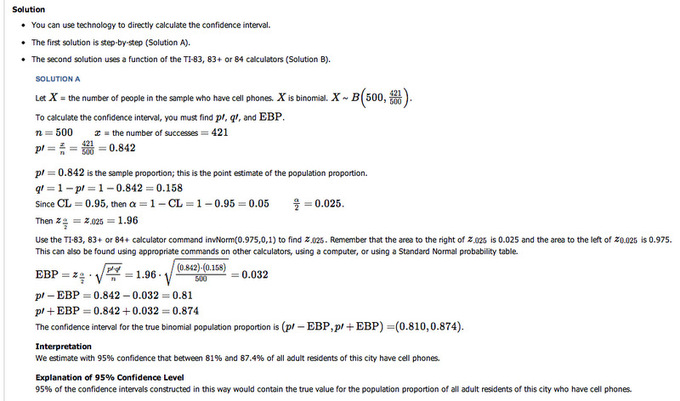Solution: This image shows the solution to our example.

## Confidence Interval for a Population Mean, Standard Deviation Known

In this section, we outline an example of finding the confidence interval for a population mean when we know the standard deviation.

### Learning Objectives

Calculate the confidence interval for a mean given that standard deviation is known

### Key Takeaways

#### Key Points

• Our example is for scores on exams in statistics that are normally distributed with an unknown population mean and a population standard deviation of 3 points.
• A random sample of 36 scores is taken and gives a sample mean (sample mean score) of 68.
• The 90% confidence interval for the mean score is $(67.1775, 68.8225)$.
• We are 90% confident that the interval from 67.1775% to 68.8225% contains the true mean score of all the statistics exams: 90% of all confidence intervals constructed in this way contain the true mean statistics exam score.

#### Key Terms

• margin of error: An expression of the lack of precision in the results obtained from a sample.
• confidence interval: A type of interval estimate of a population parameter used to indicate the reliability of an estimate.

### Step By Step Example of a Confidence Interval for a Mean—Standard Deviation Known

Suppose scores on exams in statistics are normally distributed with an unknown population mean, and a population standard deviation of 3 points. A random sample of 36 scores is taken and gives a sample mean (sample mean score) of 68. To find a 90% confidence interval for the true (population) mean of statistics exam scores, we have the following guidelines:

1. Plan: State what we need to know.
2. Model: Think about the assumptions and check the conditions.
3. State the parameters and the sampling model.
4. Mechanics: $\text{CL} = 0.90$, so $\alpha = 1-\text{CL} = 1-0.90 = 0.10$; $\alpha_{0.05}$ is $1-0.05 = 0.95$; So $\text{z}_{0.05} = 1.645$
5. Conclusion: Interpret your result in the proper context, and relate it to the original question.

1. In our example, we are asked to find a 90% confidence interval for the mean exam score, $\mu$, of statistics students.

We have a sample of 68 students.

2. We know the population standard deviation is 3. We have the following conditions:

• Randomization Condition: The sample is a random sample.
• Independence Assumption: It is reasonable to think that the exam scores of 36 randomly selected students are independent.
• 10% Condition: We assume the statistic student population is over 360 students, so 36 students is less than 10% of the population.
• Sample Size Condition: Since the distribution of the stress levels is normal, our sample of 36 students is large enough.

3. The conditions are satisfied and $\sigma$ is known, so we will use a confidence interval for a mean with known standard deviation. We need the sample mean and margin of error (ME):

$\bar { \text{x} } =68 \\ \sigma =3 \\ \text{n}=36 \\ \text{ME}={ \text{z} }_{ \frac { \alpha }{ 2 } }\left( \dfrac { \sigma }{ \sqrt { \text{n} } } \right)$

4. below shows the steps for calculating the confidence interval.

$\displaystyle {\text{ME} = 1.645\cdot \frac{3}{\sqrt{36}} = 0.8225 \\ \bar{x} - \text{ME} = 68-0.8225 = 67.1775 \\ \bar{x} + \text{ME} = 68+0.8225 = 68.8225}$

The 90% confidence interval for the mean score is $(67.1775, 68.8225)$.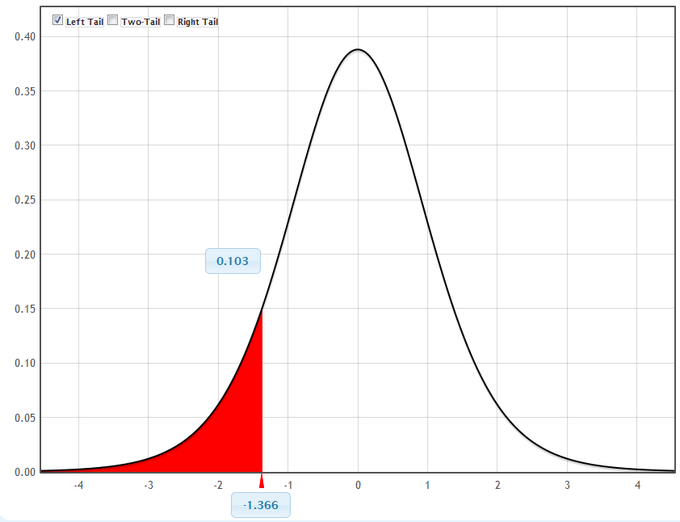Graphical Representation: This figure is a graphical representation of the confidence interval we calculated in this example.

5. In conclusion, we are 90% confident that the interval from 67.1775 to 68.8225 contains the true mean score of all the statistics exams. 90% of all confidence intervals constructed in this way contain the true mean statistics exam score.

## Confidence Interval for a Population Mean, Standard Deviation Not Known

In this section, we outline an example of finding the confidence interval for a population mean when we do not know the standard deviation.

### Learning Objectives

Calculate the confidence interval for the mean when the standard deviation is unknown

### Key Takeaways

#### Key Points

• Our example is for a study of acupuncture to determine how effective it is in relieving pain.
• We measure sensory rates for 15 random subjects, with the results being:8.6, 9.4, 7.9, 6.8, 8.3, 7.3, 9.2, 9.6, 8.7, 11.4, 10.3, 5.4, 8.1, 5.5, 6.9.
• We want to use the sample data to construct a 95% confidence interval for the mean sensory rate for the populations (assumed normal) from which we took this data.
• The 95% confidence interval for the mean score is $(7.30, 9.15)$.
• We are 95% confident that the interval from 7.30 to 9.15 contains the true mean score of all the sensory rates—95% of all confidence intervals constructed in this way contain the true mean sensory rate score.

#### Key Terms

• margin of error: An expression of the lack of precision in the results obtained from a sample.
• confidence interval: A type of interval estimate of a population parameter used to indicate the reliability of an estimate.

### Step By Step Example of a Confidence Interval for a Mean—Standard Deviation Unknown

Suppose you do a study of acupuncture to determine how effective it is in relieving pain. You measure sensory rates for 15 random subjects with the results given below:

8.6, 9.4, 7.9, 6.8, 8.3, 7.3, 9.2, 9.6, 8.7, 11.4, 10.3, 5.4, 8.1, 5.5, 6.9.

Use the sample data to construct a 95% confidence interval for the mean sensory rate for the populations (assumed normal) from which you took this data.

We have the following guidelines for such a problem:

1. Plan: State what we need to know.
2. Model: Think about the assumptions and check the conditions.
3. State the parameters and the sampling model.
4. Mechanics: $\text{CL} = 0.95$, so $\alpha = 1-\text{CL} = 1-0.95 = 0.05$. The area to the right of $\text{t}_{0.25}$ is $1-0.025 = 0.975$; so $\text{t}_{0.025, 14} = 2.14$.
5. Conclusion: Interpret your result in the proper context, and relate it to the original question.

1. In our example, we are asked to find a 95% confidence interval for the mean sensory rate, $\mu$, of acupuncture subjects. We have a sample of 15 rates. We do not know the population standard deviation.

2. We have the following conditions:

• Randomization Condition: The sample is a random sample.
• Independence Assumption: It is reasonable to think that the sensory rates of 15 subjects are independent.
• 10% Condition: We assume the acupuncture population is over 150, so 15 subjects is less than 10% of the population.
• Sample Size Condition: Since the distribution of mean sensory rates is normal, our sample of 15 is large enough.
• Nearly Normal Condition: We should do a box plot and histogram to check this. Even though the data is slightly skewed, it is unimodal (and there are no outliers) so we can use the model.

3. The conditions are satisfied and $\sigma$ is unknown, so we will use a confidence interval for a mean with unknown standard deviation. We need the sample mean and margin of error (ME).

$\overline { \text{x} } =8.2267;\text{ s}=1.6722;\text{ n}=15;$

$\text{df}=15-1=14;\text{ME}={ \text{t} }_{ \frac { \text{a} }{ 2 } }\left( \frac { 8 }{ \sqrt { \text{n} } } \right)$

4. $\text{ME} = 2.14$

$\left( \frac { 1.6722 }{ \sqrt { 15 } } \right) =0.924$

$\overline { \text{x} } =\text{ME}=8.2267-0.9240=7.3027$

$\overline { \text{x} } =\text{ME}=8.2267+0.9240=9.1507$

The 95% confidence interval for the mean score is $(7.30, 9.15)$.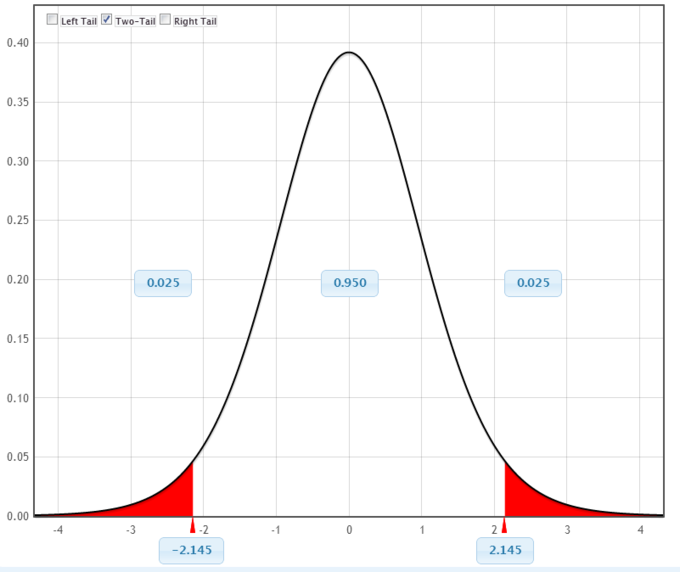Graphical Representation: This figure is a graphical representation of the confidence interval we calculated in this example.

5. We are 95% confident that the interval from 7.30 to 9.15 contains the true mean score of all the sensory rates. 95% of all confidence intervals constructed in this way contain the true mean sensory rate score.Box Plot: This figure is a box plot for the data set in our example.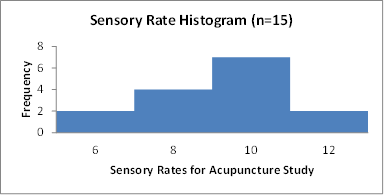Histogram: This figure is a histogram for the data set in our example.

## Estimating a Population Variance

The chi-square distribution is used to construct confidence intervals for a population variance.

### Learning Objectives

Construct a confidence interval in a chi-square distribution

### Key Takeaways

#### Key Points

• The chi-square distribution with $\text{k}$ degrees of freedom is the distribution of a sum of the squares of $\text{k}$ independent standard normal random variables.
• The chi-square distribution enters all analyses of variance problems via its role in the $\text{F}$-distribution, which is the distribution of the ratio of two independent chi-squared random variables, each divided by their respective degrees of freedom.
• To form a confidence interval for the population variance, use the chi-square distribution with degrees of freedom equal to one less than the sample size: $\text{d.f.} = \text{n}-1$.

#### Key Terms

• chi-square distribution: With $\text{k}$ degrees of freedom, the distribution of a sum of the squares of $\text{k}$ independent standard normal random variables.
• degree of freedom: Any unrestricted variable in a frequency distribution.

In many manufacturing processes, it is necessary to control the amount that the process varies. For example, an automobile part manufacturer must produce thousands of parts that can be used in the manufacturing process. It is imperative that the parts vary little or not at all. How might the manufacturer measure and, consequently, control the amount of variation in the car parts? A chi-square distribution can be used to construct a confidence interval for this variance.

The chi-square distribution with a $\text{k}$ degree of freedom is the distribution of a sum of the squares of $\text{k}$ independent standard normal random variables. It is one of the most widely used probability distributions in inferential statistics (e.g., in hypothesis testing or in construction of confidence intervals). The chi-squared distribution is a special case of the gamma distribution and is used in the common chi-squared tests for goodness of fit of an observed distribution to a theoretical one, the independence of two criteria of classification of qualitative data, and in confidence interval estimation for a population standard deviation of a normal distribution from a sample standard deviation. In fact, the chi-square distribution enters all analyses of variance problems via its role in the $\text{F}$-distribution, which is the distribution of the ratio of two independent chi-squared random variables, each divided by their respective degrees of freedom.

The chi-square distribution is a family of curves, each determined by the degrees of freedom. To form a confidence interval for the population variance, use the chi-square distribution with degrees of freedom equal to one less than the sample size:

$\text{d.f.} = \text{n}-1$

There are two critical values for each level of confidence:

1. The value of ${ \text{X} }_{ \text{R} }^{ 2 }$represents the right-tail critical value.
2. The value of ${ \text{X} }_{ \text{L} }^{ 2 }$represents the left-tail critical value.

### Constructing a Confidence Interval

As example, imagine you randomly select and weigh 30 samples of an allergy medication. The sample standard deviation is 1.2 milligrams. Assuming the weights are normally distributed, construct 99% confidence intervals for the population variance and standard deviation.

The areas to the left and right of ${ \text{X} }_{ \text{R} }^{ 2 }$and left of ${ \text{X} }_{ \text{L} }^{ 2 }$ are:

Area to the right of ${ \text{X} }_{ \text{R} }^{ 2 } = \frac{1-0.99}{2} = 0.005$

Area to the left of ${ \text{X} }_{ \text{L} }^{ 2 } = \frac{1+0.99}{2} = 0.995$

Using the values $\text{n}=30$, $\text{d.f.} = 29$ and $\text{c}=0.99$, the critical values are 52.336 and 13.121, respectively. Note that these critical values are found on the chi-square critical value table, similar to the table used to find $\text{z}$-scores.

Using these critical values and $\text{s}=1.2$, the confidence interval for $\text{s}^2$ is as follows:

Right endpoint:

$\displaystyle \frac{(\text{n}-1)\text{s}^2}{\chi_\text{L}^2} = \frac{(30-1)(1.2)^2}{13.121} \approx 3.183$

Left endpoint:

$\displaystyle \frac{(\text{n}-1)\text{s}^2}{\chi_\text{R}^2} = \frac{(30-1)(1.2)^2}{52.336} \approx 0.798$

So, with 99% confidence, we can say that the population variance is between 0.798 and 3.183.